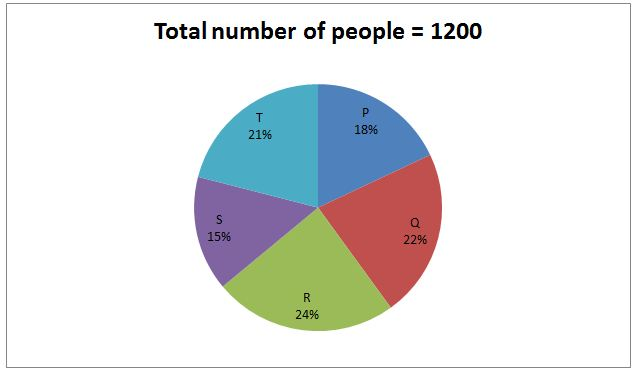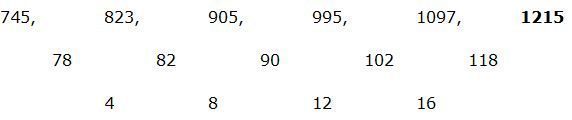# SBI Clerk prelims Quantitative Aptitude Questions 2019 (Day-01)

Quantitative aptitude is one of the most focusing subjects among banking aspirants.In SBI Clerk Prelims there are 35 Marks allotted for this section. The Marks itself shows how weightage has given for Quantitative aptitude section. The SBI Clerk 2019 exam is approaching very shortly. The exam tentatively scheduled in June 2019. Understand the importance of time and use it in the right way. As we know that SBI aptitude test questions are easy but difficult to solve within the given time limit. So practice the only solution to perform well at the time of examination. Keeping all the things in mind we are giving attention for SBI Clerk Prelims Quantitative Aptitude section. Here you can get SBI Clerk Prelims Quantitative Aptitude Questions and answer on a daily basis. SBI Clerk Prelims Quantitative Aptitude Quiz will enhance your sped and accuracy in a self-directed way.

[WpProQuiz 6111]

Directions (Q. 1 – 5): What value should come in place of (?) in the following number series?

1) 12, 16, 41, 139, 581, ?

a) 2941

b) 1853

c) 1527

d) 2675

e) 3155

2) 745, 823, 905, 995, 1097, ?

a) 1215

b) 1372

c) 1348

d) 1483

e) 1289

3) 113, 56, 57, 84, 170, ?

a) 325.5

b) 387

c) 422.5

d) 516

e) 458.5

4)  1172, 1177, 1202, 1327, ?

a) 1564

b) 1952

c) 2348

d) 2696

e) None of these

5) 288, 156, 88, 52, 32, ?

a) 36

b) 32

c) 24

d) 28

e) 20

Directions (Q. 6 – 10): Study the following information carefully and answer the given questions:

The following pie chart shows the percentage distribution of books bought by the people in 5 different stores in December 2018. Some males and females bought those books.6) If the number of males who bought the books in Store T is 34 more than the number of females who bought the books in Store T, then find the total number of females who bought the books in store T?

a) 109

b) 123

c) 117

d) 132

e) None of these

7) If the total number of people bought the books from shop A is 20 % more than the total number of people bought the books from shop S, find the total number of people bought the books from shop A and Q together?

a) 560

b) 640

c) 480

d) 720

e) None of these

8) Find the central angle for the store Q?

a) 64.5°

b) 79.2°

c) 83.6°

d) 56.8°

e) None of these

9) If in the month of February 2019, the total number of people bought the books from all the given book stores is increased by 25 % while comparing with the month of December 2018 and the percentage distribution in Feb 2019 is same as Dec 2018, then find the total number of people bought books in store P and S together in the month of Feb 2019?

a) 418

b) 526

c) 573

d) 495

e) None of these

10) Find the difference between the average number of people bought the books from store Q and R together and the average number of people bought the books from store S and T together?

a) 60

b) 72

c) 84

d) 56

e) None of these

Directions (1-5):

The pattern is, *1 + 22, *2 + 32, *3 + 42, *4 + 52, *5 + 62,….The difference of difference is, 4, 8, 12, 16,….

The pattern is, *0.5 – 0.5, *1 + 1, *1.5 – 1.5, *2 + 2, *2.5 – 2.5,….

The pattern is, +51, +52, +53, +54,….

The pattern is, ÷ 2 + 12, ÷ 2 + 10, ÷ 2 + 8, ÷ 2 + 6, ÷ 2 + 4,….

Directions (6-10):

Let the number of males and females who bought the books be x and y respectively,

Total number of people bought the books in Store T = 1200*(21/100) = 252

X + y = 252 –> (1)

X – y = 34 –> (2)

By solving the equation (1) and (2), we get,

X = 143, y = 109

The total number of females who bought the books in store T = 109

The total number of people bought the books from shop A

= > 1200*(15/100)*(120/100) = 216

The total number of people bought the books from shop Q

= > 1200*(22/100) = 264

The total number of people bought the books from shop A and Q together

= > 216 + 264 = 480

The central angle for the store Q

= > (22/100)*360 = 79.20

In the month of Feb 2019, the total number of people bought the books from all the given book stores

= > 1200*(125/100) = 1500

The total number of people bought books in store P and S together in the month of Feb 2019

= > 1500*(33/100) = 495

The average number of people bought the books from store Q and R together

= > 1200*(46/100)*(1/2) = 276

The average number of people bought the books from store S and T together

= > 1200*(36/100)*(1/2) = 216

Required difference = 276 – 216 = 60

(Or)

Difference = [(22 % + 24 %)/2] – [(15 % + 21 %)/2]

= > (46 %/2) – (36 %/2) = 5 %

Required answer = 5 % of 1200 = (5/100)*1200 = 60